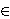### Christina Karolus

Department of Mathematics, University of Salzburg, 5020 Salzburg, Austria
e-mail: christina.karolus@sbg.ac.at

Abstract.   Let f, g, hℂ [x] be non-constant complex polynomials satisfying f(x)=g(h(x)) and let f be lacunary in the sense that it has at most l non-constant terms. Zannier proved in  that there exists a function B1(l) on , depending only on l and with the property that h(x) can be written as the ratio of two polynomials having each at most B1(l) terms. Here, we give explicit estimates for this function or, more precisely, we prove that one may take for instance

B1(l)=(4l)(2l)(3l)l+1.
Moreover, in the case l=2, a better bound is obtained using the same strategy.

2010 Mathematics Subject Classification.   11C08, 11R09

Key words and phrases.   Decomposable polynomials, lacunary polynomials

Full text (PDF) (access from subscribing institutions only)

DOI: 10.3336/gm.54.1.02

References:

1. D. Dona, On lacunary polynomials and a generalization of Schinzel's conjecture, Master thesis, Concordia University/Université de Bordeaux, 2015.

2. C. Fuchs and L. Hajdu, 30 years of collaboration, Period. Math. Hung. 74 (2017), 255-274.
MathSciNet     CrossRef

3. C. Fuchs, V. Mantova and U. Zannier, On fewnomials, integral points and a toric version of Bertini's theorem J. Amer. Math. Soc. 31 (2018), 107-134.
MathSciNet     CrossRef

4. C. Fuchs and A. Pethö , Composite rational functions having a bounded number of zeros and poles, Proc. Amer. Math. Soc. 139 (2011), 31-38.
MathSciNet     CrossRef

5. C. Fuchs and U. Zannier, Composite rational functions expressible with few terms, J. Eur. Math. Soc. 14 (2012), 175-208.
MathSciNet     CrossRef

6. A. Pethö and S. Tengely, On composite rational functions, in: Number theory, analysis, and combinatorics, De Gruyter Proc. Math., De Gruyter, Berlin, 2014, 241-259.
MathSciNet

7. A. Schinzel, On the number of terms of a power of a polynomial, Acta Arith. 49 (1987), 55-70.
MathSciNet     CrossRef

8. U. Zannier, On the number of terms of a composite polynomial, Acta Arith. 127 (2007), 157-167.
MathSciNet     CrossRef

9. U. Zannier, On composite lacunary polynomials and the proof of a conjecture of Schinzel, Invent. Math. 174 (2008), 127-138.
MathSciNet     CrossRef

10. U. Zannier, Addendum to the paper: On the number of terms of a composite polynomial, Acta Arith. 140 (2009), 93-99.
MathSciNet     CrossRef